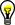# Configuring the Particle Classifier Options

Use the Particle Classifier Options tab to indicate whether the classification is Scale Dependent or Mirror Dependent.

1. Enable the Scale Dependent option if you want to distinguish samples of different sizes.
2. Enter the numeric scale factor value (between 0 and 1000) in the Scale Factor control. If the scale factor value is 0, the samples are classified independent of scale.
3. Enable the Mirror Dependent option if you want to classify mirrored objects in separate classes.
4. Enter the numeric mirror factor value (between 0 and 1000) in the Mirror Factor control. If the mirror factor value is 0, the samples are classified independent of mirror symmetry.Tip  If the samples in the ROI are not classified as you expect them to be, experiment with settings on the Preprocessing and Engine Options tabs to improve the classification.